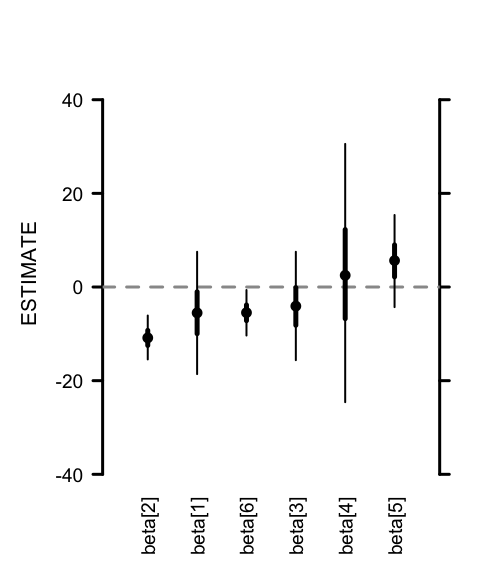MCMCvis package

# ‘MCMCvis’ package

CRAN page

Github repository

This package can be cited as: Youngflesh, C. 2018. MCMCvis: Tools to visualize, manipulate, and summarize MCMC output. Journal of Open Source Software, 3(24), 640.

## Intro

MCMCvis is an R package available on CRAN, used to visualize, manipulate, and summarize MCMC output. MCMC output may be derived from Bayesian model output fit with JAGS, Stan, or other MCMC samplers.

The package contains five functions:

• MCMCsummary - summarize MCMC output for particular parameters of interest
• MCMCpstr - summarize MCMC output for particular parameters of interest while preserving parameter structure
• MCMCtrace - create trace and density plots of MCMC chains for particular parameters of interest
• MCMCchains - easily extract posterior chains from MCMC output for particular parameters of interest
• MCMCplot - create caterpillar plots from MCMC output for particular parameters of interest

MCMCvis was designed to perform key functions for MCMC analysis using minimal code, in order to free up time/brainpower for interpretation of analysis results. Functions support simple and straightforward subsetting of model parameters within the calls, and produce presentable and ‘publication-ready’ output.

All functions in the package accept stanfit objects (created with the rstan package), mcmc.list objects (created with the rjags or coda packages), R2jags output (created with the R2jags package), jagsUI output (created with the jagsUI package), and matrices of MCMC output (one chain per column - columns to be named with parameter names). The functions automatically detect the object type and proceed accordingly. Output objects can be inserted directly into the MCMCvis functions as an argument.

#### JAGS model

library(rjags)

#create JAGS model
mf <- "
model {
for (i in 1:10)
{
y[i] ~ dnorm(mu, 0.01);
}
mu ~ dnorm(0, 0.01)
}
"

data <- list(y = rnorm(10))

jm <- rjags::jags.model(textConnection(mf),
data = data,
n.chains = 3)

jags_out <- rjags::coda.samples(jm,
variable.names = 'mu',
n.iter = 10)
library(MCMCvis)
#plug object directly into package function
MCMCsummary(jags_out)
##     mean   sd  2.5%   50% 97.5% Rhat
## mu -0.98 2.32 -5.45 -0.91  2.82 1.04

#### Stan model

library(rstan)

#create Stan model

sm <- "
data {
real y;
}
parameters {
real mu;
}
model {
for (i in 1:10)
{
y[i] ~ normal(mu, 10);
}
mu ~ normal(0, 10);
}
"

stan_out <- stan(model_code = sm,
data = data,
iter = 5)
#plug object directly into package function
MCMCsummary(stan_out)
##       mean   sd  2.5%   50% 97.5% Rhat
## mu    1.69 2.85 -3.43  1.38  5.03 1.54
## lp__ -0.62 0.51 -1.45 -0.62 -0.09 2.77

## MCMCsummary

MCMCsummary is used to output summary information from MCMC output. Two decimal places are reported by default. This can be changed using the digits argument. We’ll use the build in mcmc.list object data for the examples below, but model output of any of the supported types will behave in the same way.

data(MCMC_data)

MCMCsummary(MCMC_data)
##            mean    sd   2.5%    50% 97.5% Rhat
## alpha -11.50  7.96 -27.00 -11.54  4.39    1
## alpha -13.04  5.32 -23.60 -13.04 -2.56    1
## alpha  -9.52  1.80 -13.09  -9.53 -6.02    1
## alpha -10.70  2.18 -14.99 -10.70 -6.40    1
## alpha -11.87  4.03 -19.61 -11.87 -4.04    1
## alpha  -8.85 10.09 -28.45  -8.90 10.83    1
## beta   -5.53  6.68 -18.59  -5.52  7.52    1
## beta  -10.86  2.37 -15.46 -10.86 -6.09    1
## beta   -4.10  5.94 -15.62  -4.08  7.52    1
## beta    2.68 13.99 -24.59   2.50 30.56    1
## beta    5.60  5.07  -4.29   5.65 15.38    1
## beta   -5.48  2.48 -10.36  -5.47 -0.64    1

Specific parameters can be specified to subset summary information. Square brackets are ignored by default. For instance, all alpha parameters can be plotted using params = 'alpha'.

MCMCsummary(MCMC_data,
params = 'alpha')
##            mean    sd   2.5%    50% 97.5% Rhat
## alpha -11.50  7.96 -27.00 -11.54  4.39    1
## alpha -13.04  5.32 -23.60 -13.04 -2.56    1
## alpha  -9.52  1.80 -13.09  -9.53 -6.02    1
## alpha -10.70  2.18 -14.99 -10.70 -6.40    1
## alpha -11.87  4.03 -19.61 -11.87 -4.04    1
## alpha  -8.85 10.09 -28.45  -8.90 10.83    1

Individual parameters can also be specified. For example, one alpha (of many) may be specified. In this case, the square brackets should not be ignored, so that only the alpha parameter can be specified. Use the argument ISB = FALSE to specify particular parameters that contain brackets. ISB is short for ‘Ignore Square Brackets’. When ISB = FALSE, the params argument reads like a regular expression. Because of this, the square brackets must be escaped with \\. All other regular expression syntax is accepted, as typically applied in R. A useful cheatsheet for regular expressions in R can be found here. \\d can be used to specify any digits in a particular place.

MCMCsummary(MCMC_data,
params = 'alpha\$1\$',
ISB = FALSE)
##           mean   sd 2.5%    50% 97.5% Rhat
## alpha -11.5 7.96  -27 -11.54  4.39    1

The excl argument can be used to exclude any parameters. This can be used in conjunction with the params argument. This is particularly useful when specifying ISB = FALSE. For instance, if all alpha parameters are desired except for alpha, params = 'alpha', excl = 'alpha\$1\$', ISB = FALSE can be used. Once again, since the params argument takes a regular expression, the square brackets must be escaped using \\. When ISB = TRUE, an exact match of the specified parameter is required (excluding the square brackets). When ISB = FALSE, partial names will be matched. Leaving the default (ISB = TRUE) is generally recommended for simplicity. These arguments can be used in any of the functions in the package.

MCMCsummary(MCMC_data,
params = 'alpha',
excl = 'alpha\$1\$',
ISB = FALSE)
##            mean    sd   2.5%    50% 97.5% Rhat
## alpha -13.04  5.32 -23.60 -13.04 -2.56    1
## alpha  -9.52  1.80 -13.09  -9.53 -6.02    1
## alpha -10.70  2.18 -14.99 -10.70 -6.40    1
## alpha -11.87  4.03 -19.61 -11.87 -4.04    1
## alpha  -8.85 10.09 -28.45  -8.90 10.83    1

Setting the Rhat and n.eff arguments to FALSE can be used to avoid calculating the Rhat statistic and number of effective samples, respectively (default for Rhat and n.eff are TRUE and FALSE, respectively). Specifying FALSE can greatly increase function speed with very large mcmc.list objects. Values for Rhat near 1 suggest convergence (Brooks and Gelman 1998). Kruschke (2014) recommends n.eff > 10,000 for reasonably stable posterior estimates when using JAGS or BUGS. Substantially fewer effective samples are needed when using Stan due to the algorithm used.

MCMCsummary(MCMC_data,
params = 'alpha',
Rhat = TRUE,
n.eff = TRUE)
##            mean    sd   2.5%    50% 97.5% Rhat n.eff
## alpha -11.50  7.96 -27.00 -11.54  4.39    1 10714
## alpha -13.04  5.32 -23.60 -13.04 -2.56    1 10500
## alpha  -9.52  1.80 -13.09  -9.53 -6.02    1 10500
## alpha -10.70  2.18 -14.99 -10.70 -6.40    1 10500
## alpha -11.87  4.03 -19.61 -11.87 -4.04    1 10500
## alpha  -8.85 10.09 -28.45  -8.90 10.83    1 10500

The func argument can be used to return metrics of interest not already returned by default for MCMCsummary. Input is a function to be performed on posteriors for each specified parameter. Values returned by function will be displayed as a column in the summary output (or multiple columns if the function returns more than one value). In this way, functions from other packages can be used to derive metrics of interest on posterior output. Column name(s) for function output can be specified with the func_name argument.

MCMCsummary(MCMC_data,
params = 'alpha',
Rhat = TRUE,
n.eff = TRUE,
func = function(x) quantile(x, probs = c(0.01, 0.99)),
func_name = c('1%', '99%'))
##            mean    sd   2.5%    50% 97.5% Rhat n.eff     1%   99%
## alpha -11.50  7.96 -27.00 -11.54  4.39    1 10714 -29.58  7.04
## alpha -13.04  5.32 -23.60 -13.04 -2.56    1 10500 -25.57 -0.82
## alpha  -9.52  1.80 -13.09  -9.53 -6.02    1 10500 -13.75 -5.41
## alpha -10.70  2.18 -14.99 -10.70 -6.40    1 10500 -15.83 -5.71
## alpha -11.87  4.03 -19.61 -11.87 -4.04    1 10500 -21.09 -2.42
## alpha  -8.85 10.09 -28.45  -8.90 10.83    1 10500 -31.80 14.80

## MCMCpstr

MCMCpstr is used to output summary information from MCMC output while preserving the original structure of the specified parameters (i.e., scalar, vector, matrix, array). Function outputs a list with calculated values for each specified parameter, similar to output obtained when fitting models with the jags.samples function (as opposed to coda.samples) from the rjags package. Preserving the original structure can be helpful when plotting or summarizing parameters with multidimensional structure. Particular parameters of interest can be specified as with other functions with the params argument.

The function calculates summary information only for the specified function. The function to be used is specified using the func argument.

MCMCpstr(MCMC_data,
params = 'alpha',
func = mean)
## $alpha ##  -11.503314 -13.043566 -9.524774 -10.702370 -11.866841 -8.853045 Custom functions can be specified as well. MCMCpstr(MCMC_data, func = function(x) quantile(x, probs = 0.01)) ##$alpha
##  -29.58186 -25.57373 -13.74842 -15.83454 -21.08593 -31.79806
##
## \$beta
##  -21.097729 -16.298289 -17.637746 -29.384624  -6.153668 -11.147149

## MCMCtrace

MCMCtrace is used to create trace and density plots for MCMC output. This is useful for diagnostic purposes. Particular parameters can also be specified, as with MCMCsummary. Output is written to PDF by default to enable more efficient review of posteriors - this also reduces computation time. PDF output is particularly recommended for large numbers of parameters. pdf = FALSE can be used to prevent output to PDF.

MCMCtrace(MCMC_data,
params = c('beta\$1\$', 'beta\$2\$', 'beta\$3\$'),
ISB = FALSE,
pdf = FALSE)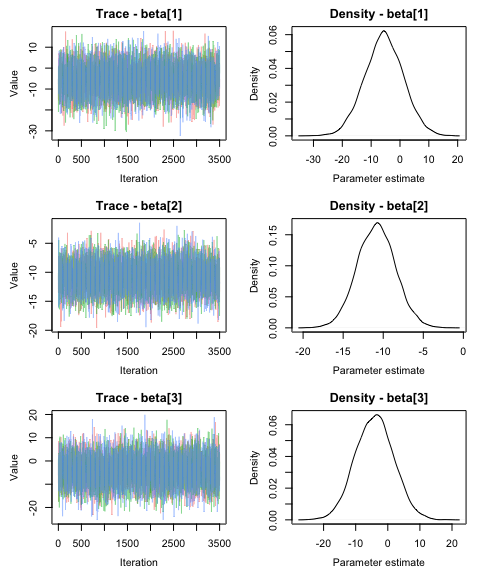PDF document will be output to the current working directory by default, but another directory can be specified. The open_pdf argument can be used to prevent the produced pdf from opening in a viewer once generated.

MCMCtrace(MCMC_data,
pdf = TRUE,
open_pdf = FALSE,
filename = 'MYpdf',
wd = 'DIRECTORY_HERE')

iter denotes how many iterations should be plotted for the chain the trace and density plots. The default is 5000, meaning that the last 5000 iterations of each chain are plotted. Remember, this is the final posterior chain, not including the specified burn-in (specified when the model was run). If less than 5000 iterations are run, the full number of iterations will be plotted.

MCMCtrace(MCMC_data,
params = c('beta\$1\$', 'beta\$2\$', 'beta\$3\$'),
ISB = FALSE,
iter = 100,
ind = TRUE,
pdf = FALSE)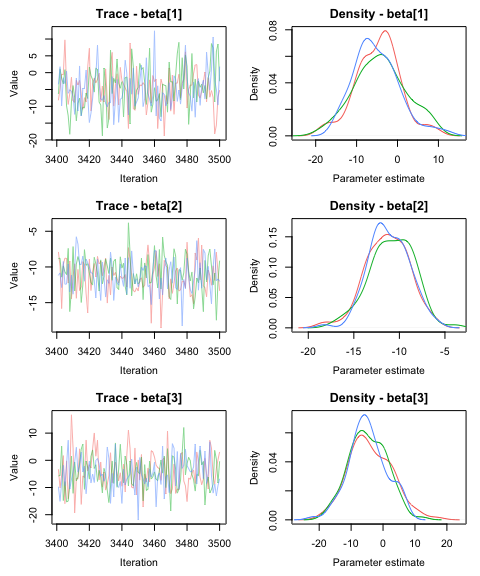If simulated data was used to fit the model, the generating values used to simulate the data can be specified using the gvals argument. This makes it possible to compare posterior estimates with the true parameter values. Generating values will be displayed as vertical dotted lines. Similar to the priors argument, if one value is specified when more than one parameter is used, this one generating value will be used for all parameters. If the lines are not apparent in the

#generating values for each parameter used to simulate data
GV <- c(-10, -5.5, -15)
MCMCtrace(MCMC_data,
params = c('beta\$1\$', 'beta\$2\$', 'beta\$3\$'),
ISB = FALSE,
gvals = GV,
pdf = FALSE)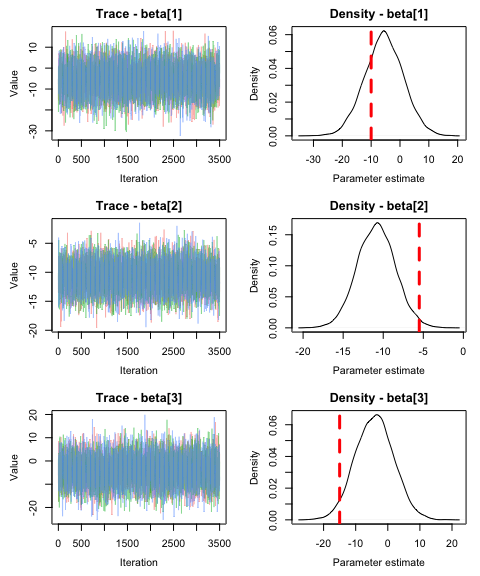ref_ovl = TRUE can be used to change how the posterior estimates are plotted based on the credible intervals. Parameters where 50% credible intervals overlap 0 are indicated by ‘open’ circles. Parameters where 50 percent credible intervals DO NOT overlap 0 AND 95 percent credible intervals DO overlap 0 are indicated by ‘closed’ grey circles. Parameters where 95 percent credible intervals DO NOT overlap 0 are indicated by ‘closed’ black circles. All median dots are represented as ‘closed’ black circles. A vertical reference at 0 is plotted by default. The position of this reference line can be modified with the ref argument. ref = NULL removes the reference line altogether.

MCMCplot(MCMC_data,
params = 'beta',
ref_ovl = TRUE)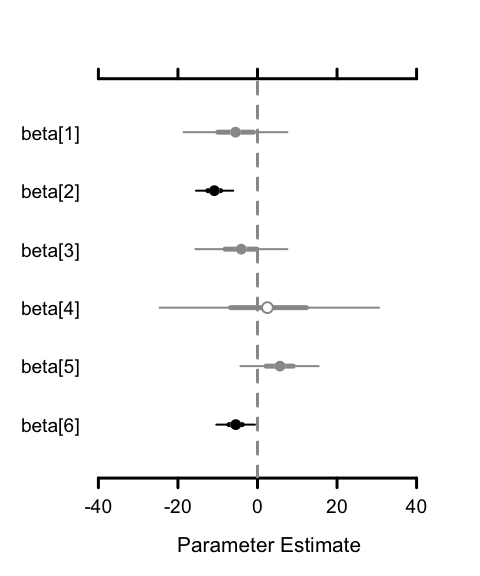The orientation of the plot can also be change using the horiz argument. ylab is then used to specify an alternative label on the ‘estimate axis’.

MCMCplot(MCMC_data,
params = 'beta',
rank = TRUE,
horiz = FALSE,
ylab = 'ESTIMATE')